Geometrization of matter and antimatter through
coadjoint action of a group on its momentum space. 2 :

Geometrical description of Dirac's antimatter

Jean-Pierre Petit & Pierre Midy
Observatoire de Marseille

Abstract :

...vWe extend the precedent group to a four-components orthochron set. This operation gives a geometrical interpretation of antimatter after Dirac.

1) Introduction :

...In a former paper  we have presented a description of elementary particles ins a ten-dimensional space, i.e. space-time (x,y,z,t) plus six additional dimensions :

(1)

{ z 1 , z 2 , z 3 , z 4 , z 5 , z 6 }

We presented a 16-dimensions group, an extension of the Poincaré orthochron subgroup, acting on :

- its 16-dimensions momentum space

- its 10-dimensional movement space.

The six additional components of the momentum have been identified to the charges of the particles :

(2)

{ q , cB , cL , cm , ct , v }

so that the momentum becomes :

(3)

Jpe = { q , cB , cL , cm , ct , v , Jp }

where Jp represent the classical moment, from the orthochron Poincaré sub-group :

(4)

Jpo = { E , p , f , l }

after J.M.Souriau .

We have figured the link between the species of moments and the species of movement, suggesting that :

- The movement of matter corresponds to { z i > 0 } sector.

- The movement of antimatter corresponds to { z i < 0 } sector.

- The movement of photons corresponds to { z i = 0 } plane.

All that must be now justified.

2) Introducing a four components group. Geometrization of Dirac's antimatter.

...The precedent 16-dimensional group had two components, correspondong to the two orthochron components of the Lorentz group, Ln ( neutral component ) and Ls , with :

(5)

Lo ( orthochron sub-group ) = Ln U Ls

Our group was an extension of the orthochron Poincaré sub-group :

(6)

Go = Gn U Gs

and we wrote it :

(7)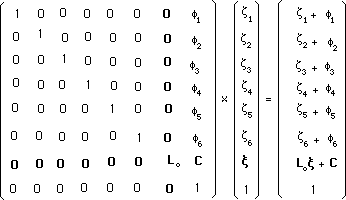The corresponding coadjoint action was :

(8)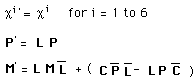with :

(9)

{c i} = { q , cB , cL , cm , ct , v }

...In such a group no element transforms the movement of a matter mass-point into the movement of an antimatter mass-point, and vice versa. According to the chosen definition of antimatter, through a :

(10)

z - Symmetry : {z i} ----> {- z i}

some element should reverse the additional dimensions. With :

(11)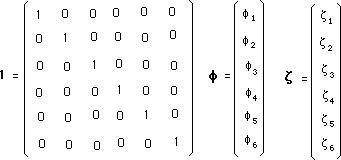we can write the precedent group into a more compact form :

(12)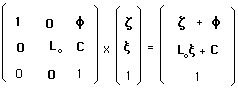It contains the neutral element :

(13)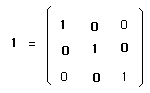The matrix that reverses the additional dimensions is be the following orthochron commuter :

(14)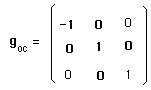We can duplicate the precedent group through the operation :

(15)

go x goc

It is equivalent to write the new four component group, whose element is :

(16)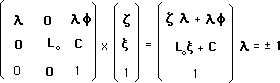The corresponding coadjoint action is :

(17)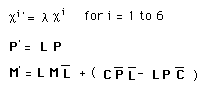We see that ( l = - 1 ) reverses the charges. In that case the inversion of the additional dimensions :

(18)

z - Symmetry : {z i} ----> {- z i}

goes with a :
(19)

C-symmetry (or charge conjugation ) :
{ q , cB , cL , cm , ct , v } ---> {- q ,- cB ,- cL ,- cm ,- ct , - v }

which corresponds to Dirac's description of antimatter , so that the present paper represents a geometrization of antimatter after Dirac.